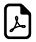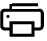# Parts of Multiplication Equations## Terminology in Multiplication

Operations Vocabulary Explanation Example
Multiplication Multiplicand Number which is multiplied by another numberMultiplier Number with which multiply is called the multiplier
Product Product is the result of multiplying.

## Basic Math Terms Used In Multiplication:

5 x 3 = 15 ,

Here, 5 is Multiplicand

3 is Multiplier

15 is Product

### Multiplication Example2:

3 x 3 = 9,

Here, 3 is Multiplicand

3 is Multiplier

9 is Product

###### These vocabulary of multiplication is the basic things in general mathematics